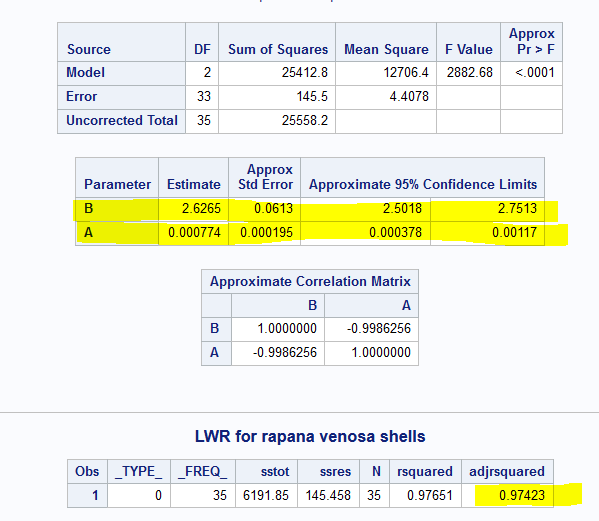## Estimating parameters for each group separately (multiple group data)

Hi,
I need to estimate parameters a and b as well as R-squared from data for each group (expno=1, expno=2, expno=3 etc.,) separately at once. Could someone help me to modify the below codes? Many thanks

``````FILENAME CSV "/folders/myfolders/umar2021.csv" TERMSTR=CRLF;

PROC IMPORT DATAFILE=CSV
OUT=umar
DBMS=CSV
REPLACE;
RUN;
title 'LWR for shells';
data exp;
set umar;
if expno=1 or expno=2 or expno=3;
run;

proc nlin data=exp method=marquardt;
parms B=3 A=0.01;

model w=A*tl**B;

output out=expp p=pw r=w_residual;
run;
proc summary data=expp;
var w_residual w;
output out=stats css(w)=sstot uss(w_residual)=ssres N=N;
run;
data expp;
set stats;
rsquared=1-(ssres/sstot);
adjrsquared = 1-(1-rsquared)*(N-1) / (N- 3  -1);
run;
proc print data=expp;
run; ``````

1 ACCEPTED SOLUTION

Accepted Solutions

## Re: Estimating parameters for each group separately (multiple group data)

Thanks, I got the desired results using these codes. Also, the error related to import was also corrected following your suggestions. I attached the SAS output results.

PS. I also need to get plot (figures with curve line using power function) for expno 1, expno 2 and expno 3 where "tl" at x-axis and "w" at y-axis.

9 REPLIES 9PGStats
Opal | Level 21

## Re: Estimating parameters for each group separately (multiple group data)

Sort your data by expno and use a BY expno statement in proc nlin and in proc summary.

PG

## Re: Estimating parameters for each group separately (multiple group data)

Hi,
I modify my codes like as below but I did not get results separately for each group.

``````data exp;
set rapana;
by expno;
if expno=1 or expno=2 or expno=3;
run;``````

Also added in summary like this:

``````proc summary data=expp by expno;
var w_residual w;
output out=stats css(w)=sstot uss(w_residual)=ssres N=N;
run;``````

## Re: Estimating parameters for each group separately (multiple group data)

Hello,

If you get errors in the LOG it is better to post the LOG and not just the code.

Your data step will fail if the data are not previously sorted by "expno". Use PROC SORT.

And the BY statement in PROC SUMMARY should be a separate statement, thus preceded by a semicolon.

And your PROC NLIN should have the BY-statement as well.

I repeat what PROC NLIN says about its BY statement. It says much more than the below. The below is just the 1st paragraph. Specify "BY expno;".

The NLIN Procedure

BY Statement
BY variables;

You can specify a BY statement with PROC NLIN to obtain separate analyses of observations in groups that are defined by the BY variables. When a BY statement appears, the procedure expects the input data set to be sorted in order of the BY variables. If you specify more than one BY statement, only the last one specified is used.

Cheers,

Koen

## Re: Estimating parameters for each group separately (multiple group data)

Thanks for the comments and hints. I followed them like this:

``````FILENAME CSV "/folders/myfolders/rapana2021.csv" TERMSTR=CRLF;

PROC IMPORT DATAFILE=CSV
OUT=rapana
DBMS=CSV
REPLACE;
RUN;

PROC SORT DATA=exp;
BY expno;
RUN;``````

But got this error:

ERROR: Import unsuccessful. See SAS Log for details.
NOTE: The SAS System stopped processing this step because of errors.
NOTE: PROCEDURE IMPORT used (Total process time):
real time 0.54 seconds
cpu time 0.48 seconds

## Re: Estimating parameters for each group separately (multiple group data)

I need to estimate parameters a and b for each "expno group" (expno 1, 2 and 3 separately).## Re: Estimating parameters for each group separately (multiple group data)

Hello @afgdurrani0 ,

I see and I understand, but the problem is not in your NLIN-analyses.

You have a PROC IMPORT problem that needs to be solved first.

But why do you import? I see that you have run your analysis on the 3 groups together, so the import has already been done? No need for another PROC IMPORT I would say, correct?

To correct the PROC IMPORT (if still needed), go to the LOG-window and copy the data step that was generated by PROC IMPORT. Paste the data step in your SAS-editor and adjust accordingly until all errors are gone.

You can also import using a UI (User Interface). As well SAS 9.4 as VIYA have UIs to import CSV-files without code.

Cheers,

Koen

## Re: Estimating parameters for each group separately (multiple group data)

Thanks, I got the desired results using these codes. Also, the error related to import was also corrected following your suggestions. I attached the SAS output results.

PS. I also need to get plot (figures with curve line using power function) for expno 1, expno 2 and expno 3 where "tl" at x-axis and "w" at y-axis.

## Re: Estimating parameters for each group separately (multiple group data)

Hello,

With respect to the plot ...

, this example should give you the right inspiration:

SAS/STAT® 15.2 User's Guide

The NLIN Procedure
Example 87.5 Comparing Nonlinear Trends among Groups

https://go.documentation.sas.com/doc/en/statug/15.2/statug_nlin_examples05.htm

Koen

## Re: Estimating parameters for each group separately (multiple group data)

Thanks for the help 🙂
Best regards
Discussion stats
• 9 replies
• 557 views
• 6 likes
• 3 in conversation